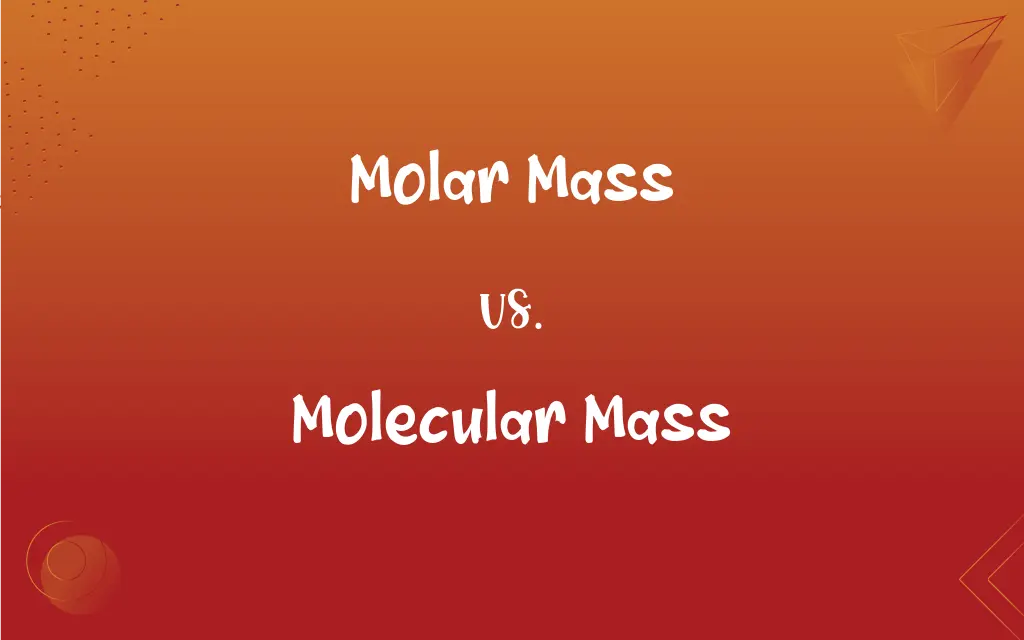# Molar Mass vs. Molecular Mass: What's the Difference?Edited by Huma Saeed || By Sawaira Riaz || Published on October 22, 2023
Molar Mass is the mass of one mole of a substance in grams/mole, while Molecular Mass is the sum of atomic masses of all atoms in a molecule in atomic mass units (amu).## Key Differences

Molar Mass is typically expressed in grams per mole (g/mol). In contrast, Molecular Mass is usually conveyed in atomic mass units (amu) or Daltons.
Molar Mass gives an average mass of all the molecules in a substance, considering isotopic abundance. Molecular Mass, however, refers to a specific molecule's total mass.
The value of Molar Mass is determined using Avogadro's number, relating it to a specific amount of substance. Molecular Mass derives its value from the periodic table, adding up individual atomic masses.
While Molar Mass can be applied to both elements and compounds, Molecular Mass is often associated with molecules, especially compounds.
Molar Mass plays a crucial role in stoichiometric calculations in chemistry, while Molecular Mass is significant when analyzing molecular structures and behaviors.

## Comparison Chart

### Unit

Grams per mole (g/mol)
Atomic mass units (amu) or Daltons

### Scale and Application

Average mass of all molecules
Mass of a specific molecule

Periodic table

### Applicable Substances

Elements and compounds
Typically molecules, especially compounds

### Role in Chemistry

Stoichiometric calculations
Analyzing molecular structures and behaviors

## Molar Mass and Molecular Mass Definitions

#### Molar Mass

The mass of one mole of a substance.
The Molar Mass of water (H₂O) is 18.015 g/mol.

#### Molecular Mass

Derived from the periodic table.
The Molecular Mass of a substance is calculated using atomic masses from the periodic table.

#### Molar Mass

Used in stoichiometric calculations in chemistry.
Molar Mass is essential for converting between grams and moles.

#### Molecular Mass

The sum of atomic masses in a molecule.
The Molecular Mass of water (H₂O) is approximately 18 amu.

#### Molar Mass

A value representing the average mass of molecules in a substance.
Carbon has a Molar Mass of 12.01 g/mol.

#### Molecular Mass

A specific value for a particular molecule.
Oxygen molecule (O₂) has a Molecular Mass of 32 amu.

#### Molar Mass

Using Avogadro's number, we can determine the Molar Mass of an element or compound.

#### Molecular Mass

Represents individual molecules rather than averages.
The Molecular Mass of methane (CH₄) is 16 amu.

#### Molar Mass

A physical property of substances.
The Molar Mass of sodium chloride (NaCl) is approximately 58.44 g/mol.

#### Molecular Mass

Often used in molecular analysis.
Knowing the Molecular Mass helps in structural determination of compounds.

## FAQs

#### How is Molar Mass related to Avogadro's number?

Molar Mass in grams of a substance contains Avogadro's number of entities.

#### Is Molar Mass the same for all isotopes of an element?

No, Molar Mass is an average considering isotopic abundance, while isotopes have distinct Molecular Masses.

#### Can the Molecular Mass be a fraction?

Yes, since it's an average based on isotopic abundance.

#### Can two compounds have the same Molecular Mass?

Yes, they can be isomers with the same Molecular Mass but different structures.

#### How does one determine the Molar Mass of a compound?

By summing up the atomic masses of its elements multiplied by their stoichiometric coefficients.

#### Does Molar Mass change with temperature or pressure?

No, Molar Mass is a constant property of a substance.

#### Does Molar Mass account for isotopes?

Yes, it's an average value considering isotopic abundance.

#### Are Molar Mass and molar volume related?

Yes, at STP, one mole of any gas occupies a molar volume of 22.4 liters.

#### Why is Molecular Mass significant in structural chemistry?

Molecular Mass can provide insights into molecular size and structure.

#### Is Molecular Mass the same as formula mass?

For covalent compounds, yes. For ionic compounds, it's more appropriate to use "formula mass."

#### Why is Molar Mass essential in laboratory work?

Molar Mass aids in converting between the amount of substance and mass.

#### Why might a molecule's Molecular Mass be different from its experimental value?

Isotopic variations and experimental errors can cause discrepancies.

#### Why is Molar Mass crucial in chemical reactions?

It helps in determining reactants' and products' quantities.

#### Can a molecule have the same Molar and Molecular Mass?

Only if the units are considered. For example, H₂O's Molecular Mass is 18 amu and its Molar Mass is 18 g/mol.

#### How is Molar Mass used in titrations?

It helps relate the volume of one solution to the mass of another reactant.

#### Is Molecular Mass always an integer?

Typically, but due to isotopic abundance, it can be fractional.

#### How does one determine the Molecular Mass of an unknown compound?

Techniques like mass spectrometry can provide precise Molecular Mass values.

#### Do isotopic variations affect Molecular Mass significantly?

Yes, especially if the isotopic abundance varies considerably.

#### Can molecules with similar Molecular Masses have different properties?

Absolutely. Structural differences can lead to varied properties.

#### Does increasing temperature affect Molecular Mass?

No, Molecular Mass is an intrinsic property of a molecule.